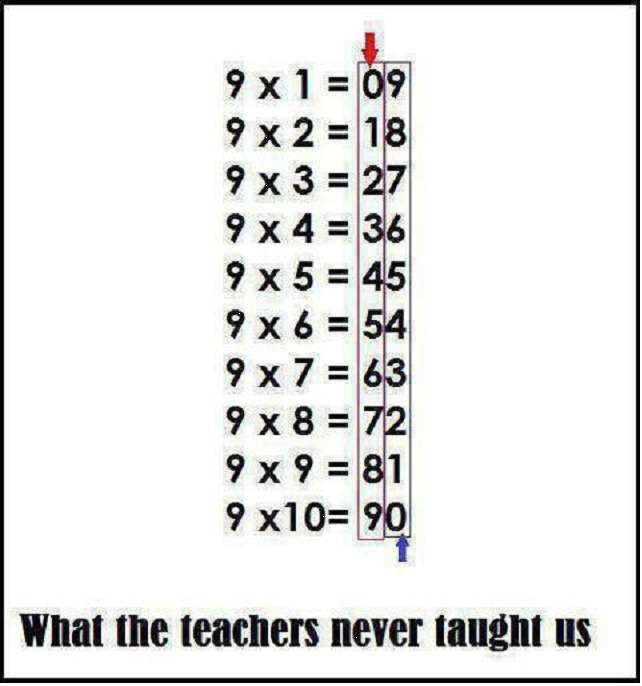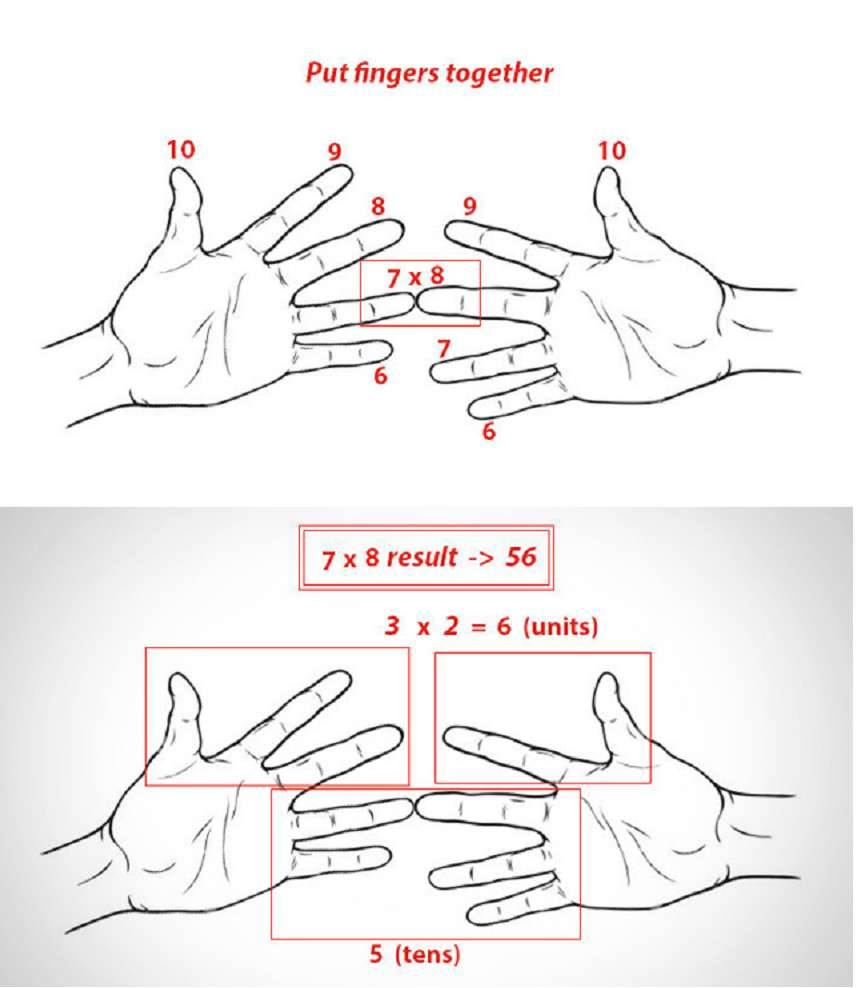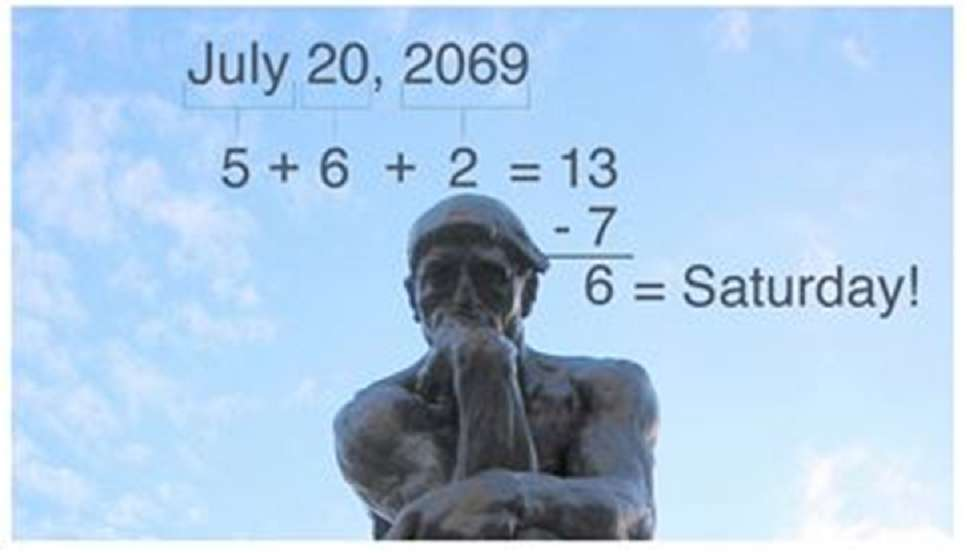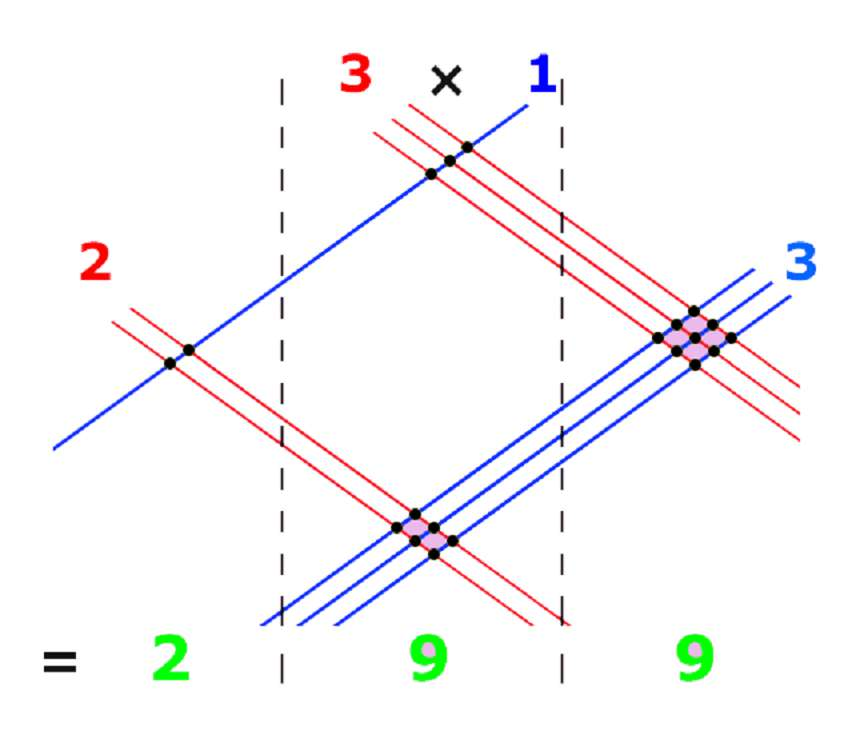## Math Hacks and Tricks: 11 Ways to Make Math Easier

For most people, math is the bane of their existence in the classroom and elsewhere. Many would even brag about their lack of proficiency in the act of making sense of two or more numbers.

It would be fine if numbers were merely a trifle, but math really does make the world go round, or at least explain why it does.

Mathematics is the language that links human understanding to the mysteries of the universe and everything else in between.

It’s also the language of money, which is perhaps why a lot of people aren’t that financially literate. In short, math is a very important part of life, whether we understand it or not.

If that has caught your attention, then perhaps you may want to actually get better with math. There isn’t a real surefire shortcut to progress and success, but there are some math hacks and tricks that can help you get better and understand the way of numbers more.

## Easier Ways to Multiply

Multiplying by 6: Multiplying the number by 3, then multiply the result by 2.

e.g. 12 x 6 = ( 12 x 3 ) x 2 = 36 x 2 = 72

Multiplying by 9: Multiply the number by 10, then subtract the original number.

e.g. 35 x 9 = ( 35 x 10 ) – 35 = 350 – 35 = 315

Multiplying by 11: Multiply the number by 10, then add the original number.

e.g. 527 x 11 = ( 527 x 10 ) + 527 = 5270 + 527 = 5797

Multiplying two-digit numbers by 11: Add the two digits together and put the result in between them (and carrying the extra digit to the higher digit if the result has two digits).

e.g. 89 x 11 → 8 (8+9) 9 → 8 (17) 9 → 8+1 (7) 9 → 979

Multiplying by 12: Multiply the number by 10, then add twice the original number.

e.g. 23 x 12 = ( 23 x 10 ) + ( 23 x 2 ) = 230 + 46 = 276

Multiplying by 13: Multiply the number by 3 and add 10 times original number.

e.g. 47 x 13 = ( 47 x 3 ) + ( 47 x 10 ) = 141 + 470 = 611

Multiplying by 14: Multiply the number by 7 and then multiply by 2.

e.g. 8 x 14 = ( 8 x 7 ) x 2 = 56 x 2 = 112

Multiplying by 15: Multiply the number by 10 and add 5 times the original number, as above.

e.g. 10 x 15 = ( 10 x 10 ) + ( 10 x 5 ) = 100 + 50 = 150

Multiplying by 16: Multiply the number by 8, then by 2.

e.g. 40 x 16 = ( 40 x 8 ) x 2 = 320 x 2 = 640

Multiplying by 17: Multiply the number by 7 and add 10 times original number.

e.g. 7 x 17 = ( 7 x 7 ) + ( 7 x 10) = 49 + 70 = 119

Multiplying by 18: Multiply the number by 20, then subtract twice the original number.

e.g. 56 x 18 = ( 56 x 20 ) – ( 56 x 2 ) = 1120 – 112 = 1008

Multiplying by 19: Multiply the number by 20, then subtract the original number.

e.g. 72 x 19 = ( 72 x 20 ) – 72 = 1440 – 72 = 1368

Multiplying by 24: Multiply the number by 8, then multiply by 3.

e.g. 32 x 24 = ( 32 x 8 ) x 3 = 256 x 3 = 768

Multiplying by 27: Multiply the number by 30, then subtract 3 times the original number.

e.g. 194 x 27 = ( 194 x 30 ) – ( 194 – 3 ) = 5820 – 582 = 5238

Multiplying by 45: Multiply the number by 50, then subtract 5 times the original number.

e.g. 67 x 45 = ( 67 x 50 ) – ( 67 x 5 ) = 3350 – 335 = 3015

Multiplying by 98: Multiply the number by 100 and subtract twice the original number.

e.g. 19 x 98 = ( 19 x 100 ) – (19 x 2 ) = 1900 – 38 = 1862

Multiplying by 99: Multiply the number by 100 and subtract the original number.

e.g. 31 x 99 = ( 31 x 100 ) – 31 = 3100 – 31 = 3069

## More Easier Ways to MultiplyAnd…## Determining what day of the week a specific date is (without memorizing or looking at a calendar)## Quick-Squaring Two-Digit Numbers Ending in 5

Multiply the first digit by itself plus 1, then put 25 at the end of it.

e.g. 552 → 5 x (5+1) → 5 x 6 → 30 → 3025

## How Japanese Children Learn Multiplication## If you’re having trouble in Trigonometry, just use SohCahToa.

This mnemonic device helps you remember how make sense of triangles. Soh means SinA=Opposite/Hypotenuse, Cah means CosA=Adjacent/Hypotenuse, and Toa means TanA=Opposite/Adjacent.

## Convert your salary to an hourly rate.

Drop the last two zeroes of your salary, then divide by two.

e.g. \$3,000 per month → \$30 / 2 = \$15 per hour

## Calculate how long it will take to triple your investment.

The Rule of 115: An odd name for a rule of thumb, but this is very useful for those who are looking to grow their money. Simply divide 115 or 110 by the growth rate, which should give you the amount of time it takes to do just that.

It’s a good rule to know how long you have with a certain investment, especially if you’re not particularly risk-averse.

e.g. 10% per year → 115 / 10 = 11.5 years

Take number X, subtract by the nearest multiple of 10 to get the difference D. You then multiply (X-D) with (X-D), then add D2 to get your answer.

It’s easier because one of the numbers you come up with is a multiple of 10, so you’re basically multiplying a double digit number with a single digit number then adding 0 at the end before you add the square of D.

e.g. 772 → D = 80 – 77 = 3 → ( 77 – 3 ) x ( 77 + 3 ) + 32 = 74 x 80 + 9 → ( 70 x 80 ) + ( 4 x 80 ) + 9 = 5600 + 320 + 9 = 5920 + 9 = 5929

It seems hard and long-winded at first, but you should be able to get the hang of it with some practice. Since you end up dealing with multiples of 10 (once you use the multiplication shortcut for multiplying a double-digit number to a multiple of 10), you should find it easier with some practice rather than just tackling 772 head on.

## Asset Allocation by Age.

Here’s another rule of thumb for your money management needs. If you don’t know how much of your assets you should put into certain investments, just remember this. Subtract your current age from 120, and the result is the percentage you should put into stocks.

e.g. 40 years old → 120 – 40 = 80% in stocks, 20% in fixed income products

Hope this list helped get you even more excited about Math. If you’re looking for more tips, you can check out more of our Math lessons.

Like the article? Share it with a friend.

# The StudyPug Blog

Unlock unlimited video lessons and practice